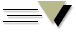## Ars Brevis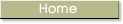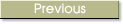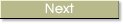###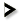11. Questions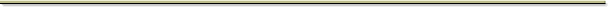###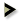9. Multiplying the Fourth Figure

9. Questions about the Multiplication of the Fourth Figure

62. Question: by what method does the intellect train itself to be general in general understanding?  Go to the multiplication of the fourth figure, where you will see how the intellect multiplies conditions with which it multiplies its objects and its understanding, to make it general to many great sciences and to cloth it with many habits. And this is enough about multiplying the fourth figure, for the sake of brevity.